Examples

Chapter 9 Class 11 Sequences and Series (Term 1)
Serial order wise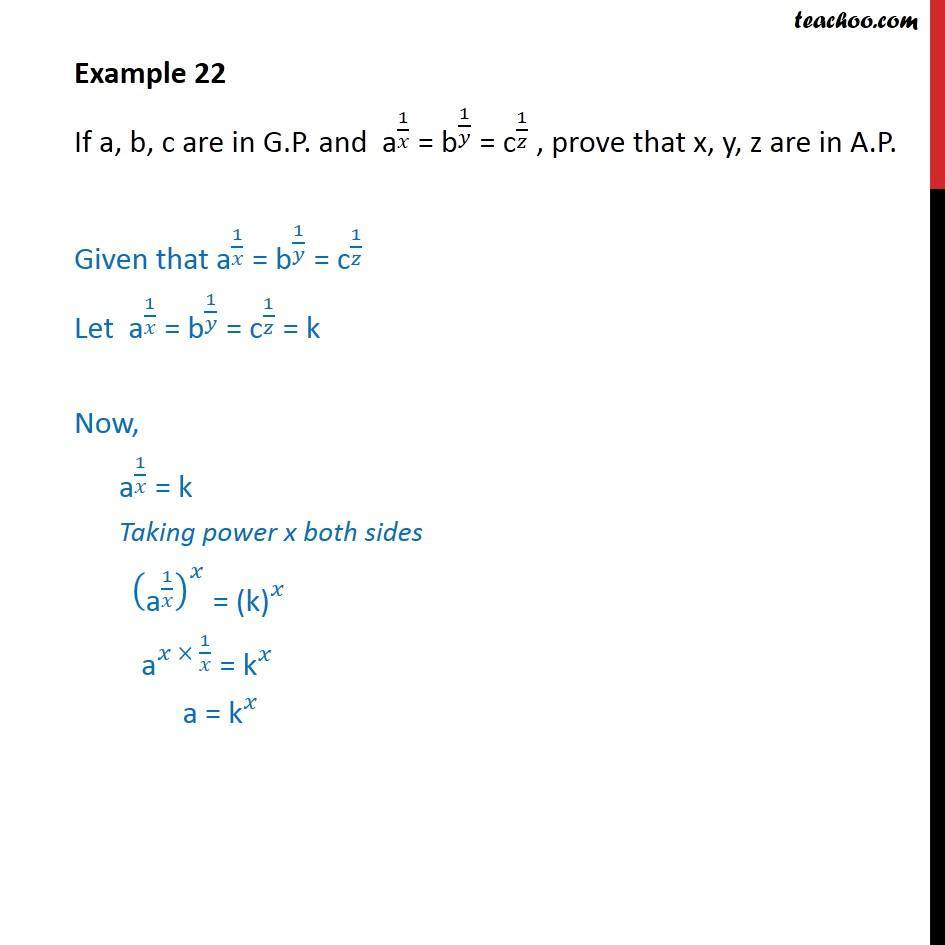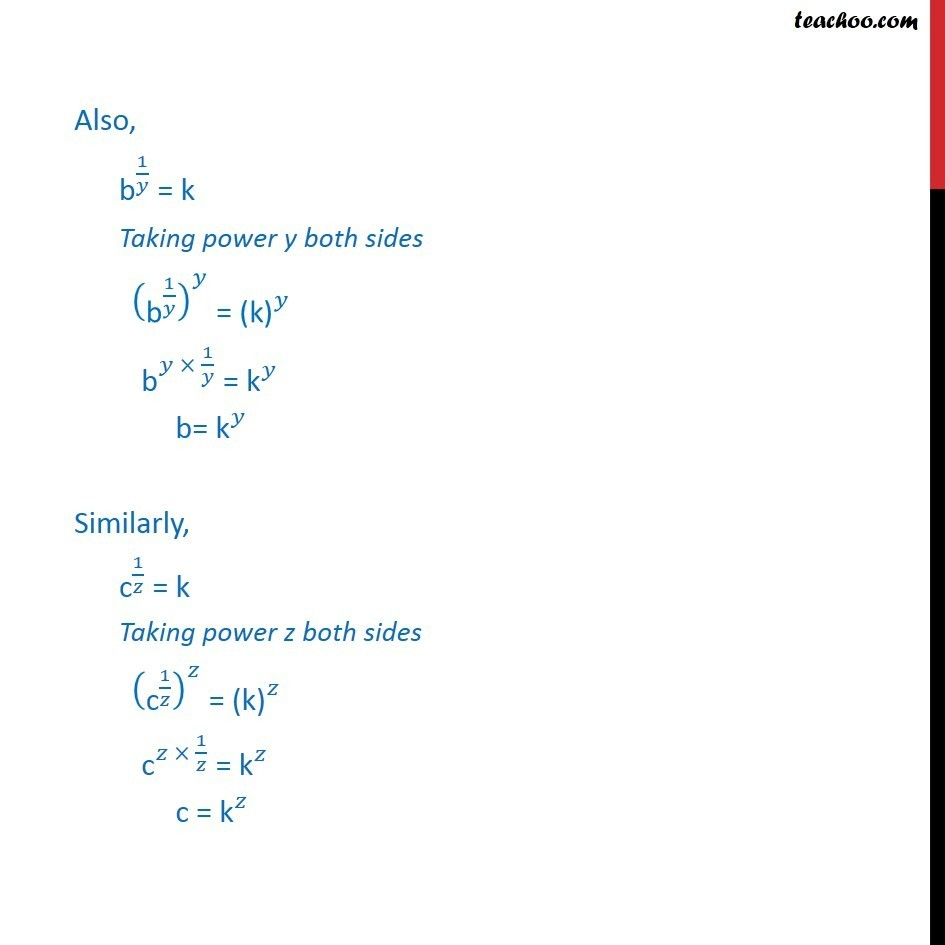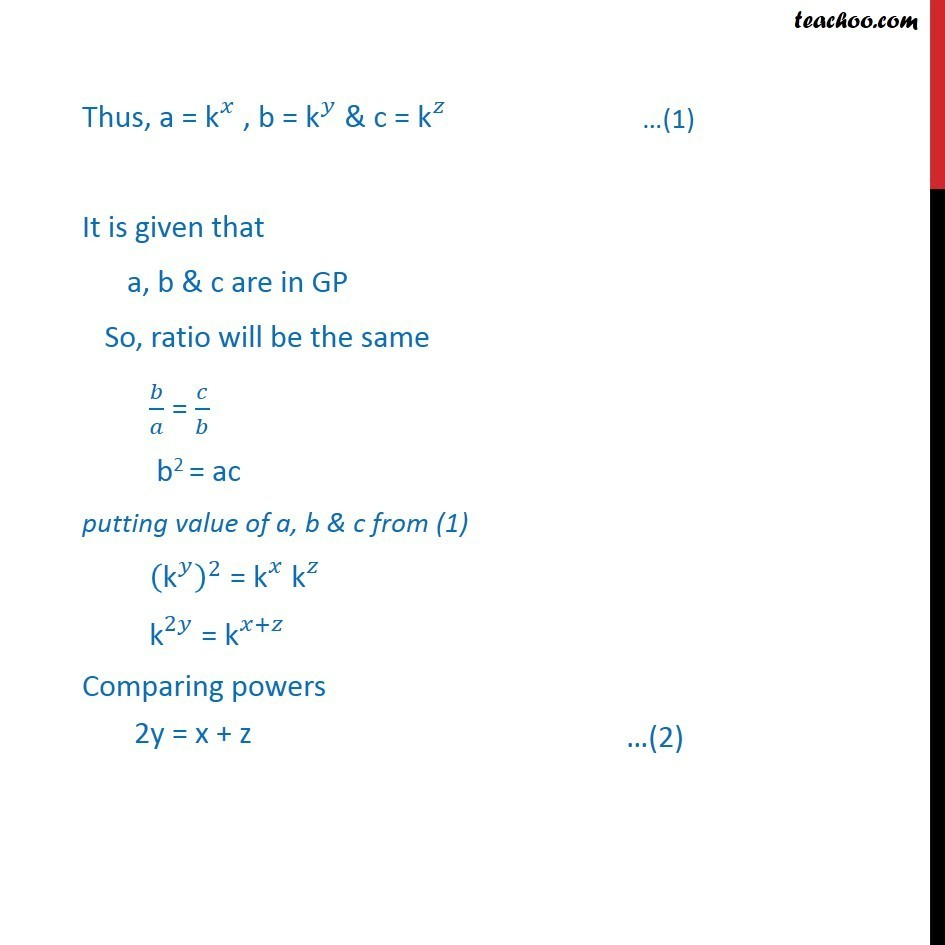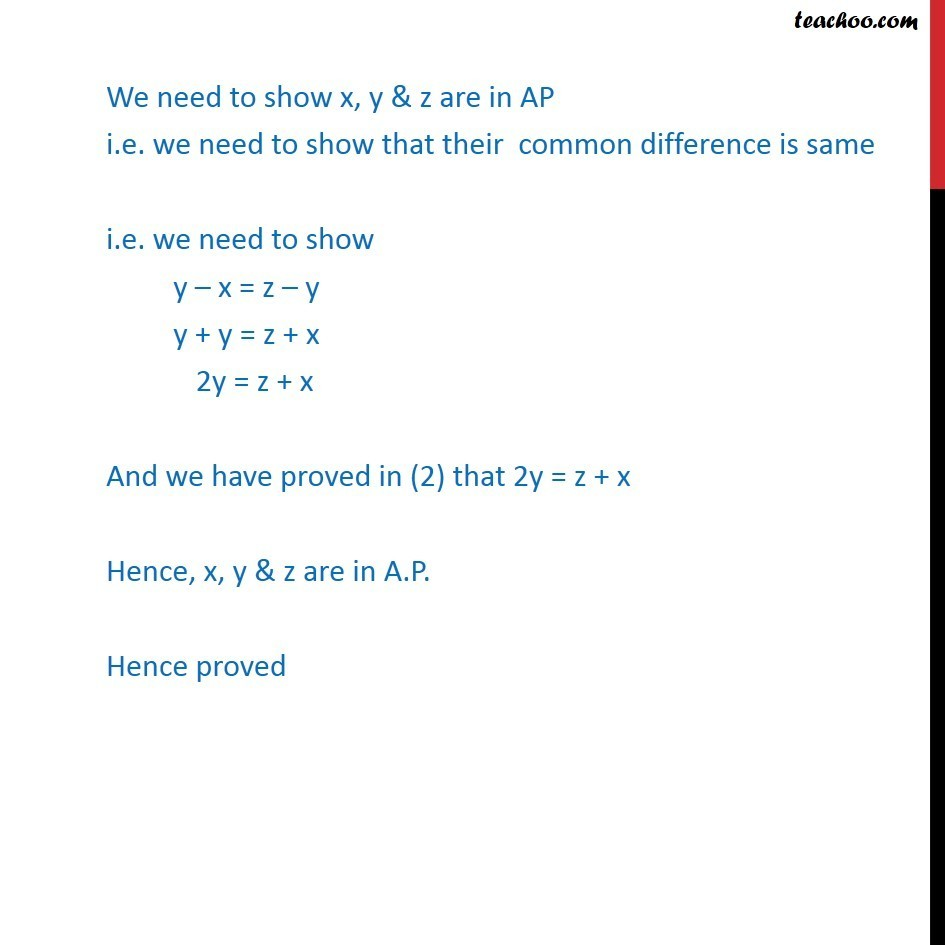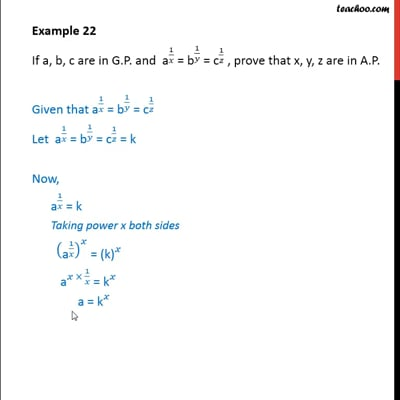This video is only available for Teachoo black users

### Transcript

Example 22 If a, b, c are in G.P. and "a" ^(1/𝑥) = "b" ^(1/𝑦) = "c" ^(1/𝑧) , prove that x, y, z are in A.P. Given that "a" ^(1/𝑥) = "b" ^(1/𝑦) = "c" ^(1/𝑧) Let "a" ^(1/𝑥) = "b" ^(1/𝑦) = "c" ^(1/𝑧) = k Now, "a" ^(1/𝑥) = k Taking power x both sides ("a" ^(1/𝑥) )^𝑥 = 〖"(k)" 〗^𝑥 "a" ^(𝑥 × 1/𝑥) = "k" ^𝑥 a = "k" ^𝑥 Also, "b" ^(1/𝑦) = k Taking power y both sides ("b" ^(1/𝑦) )^𝑦 = 〖"(k)" 〗^𝑦 "b" ^(𝑦 × 1/𝑦) = "k" ^𝑦 b= "k" ^𝑦 Similarly, "c" ^(1/𝑧) = k Taking power z both sides ("c" ^(1/𝑧) )^𝑧 = 〖"(k)" 〗^𝑧 "c" ^(𝑧 × 1/𝑧) = "k" ^𝑧 c = "k" ^𝑧 Thus, a = "k" ^𝑥 , b = "k" ^𝑦 & c = "k" ^𝑧 It is given that a, b & c are in GP So, ratio will be the same 𝑏/𝑎 = 𝑐/𝑏 b2 = ac putting value of a, b & c from (1) ("k" ^𝑦 )^2 = "k" ^𝑥 "k" ^𝑧 "k" ^2𝑦 = "k" ^(𝑥+𝑧) Comparing powers 2y = x + z We need to show x, y & z are in AP i.e. we need to show that their common difference is same i.e. we need to show y – x = z – y y + y = z + x 2y = z + x And we have proved in (2) that 2y = z + x Hence, x, y & z are in A.P. Hence proved